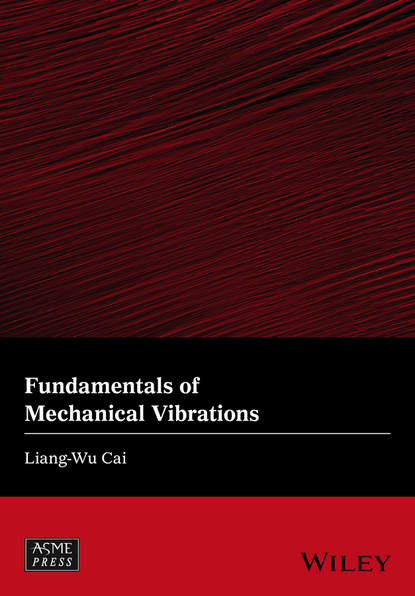Fundamentals of Mechanical Vibrations

# Fundamentals of Mechanical Vibrations

Автор
Год написания книги
2018
This introductory book covers the most fundamental aspects of linear vibration analysis for mechanical engineering students and engineers. Consisting of five major topics, each has its own chapter and is aligned with five major objectives of the book. It starts from a concise, rigorous and yet accessible introduction to Lagrangian dynamics as a tool for obtaining the governing equation(s) for a system, the starting point of vibration analysis. The second topic introduces mathematical tools for vibration analyses for single degree-of-freedom systems. In the process, every example includes a section Exploring the Solution with MATLAB. This is intended to develop student's affinity to symbolic calculations, and to encourage curiosity-driven explorations. The third topic introduces the lumped-parameter modeling to convert simple engineering structures into models of equivalent masses and springs. The fourth topic introduces mathematical tools for general multiple degrees of freedom systems, with many examples suitable for hand calculation, and a few computer-aided examples that bridges the lumped-parameter models and continuous systems. The last topic introduces the finite element method as a jumping point for students to understand the theory and the use of commercial software for vibration analysis of real-world structures.

## Читать онлайн

Авторизуйтесь чтобы можно было оставлять комментарии

## Отзывы

список сообщений пуст